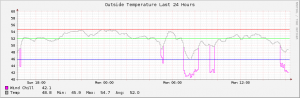# Calculating wind chill with RRDTool

In the process of switching over my Gnuplot graphs to RRD, I needed to re-do the SQL function I used for calculating wind chill into RPN notation. Quite straight forward in sql:

[code lang=”sql”]
CREATE OR REPLACE FUNCTION apparent_temp( float, float, float )
RETURNS float AS ‘
DECLARE
tempf ALIAS FOR \$1;
relh ALIAS FOR \$2;
speed ALIAS FOR \$3;
aptemp float;
BEGIN
IF tempf >= 80 AND relh >= 40 THEN
aptemp = -42.379 + 2.04901523*tempf + 10.14333127*relh
– 0.22475541*tempf*relh – 0.00683783*(tempf^2)
– 0.05481717*(relh^2) + 0.00122874*(tempf^2)*relh
+ .00085282*tempf*(relh^2) – 0.00000199*(tempf^2)*(relh^2);
ELSIF speed >= 3 AND tempf <= 50 THEN
aptemp = 35.74 + .6215*tempf – 35.75*(speed^.16)
+ .4275*tempf*(speed^.16);
ELSE
aptemp = tempf;
END IF;
RETURN aptemp;
END;
‘ LANGUAGE plpgsql IMMUTABLE STRICT;
[/code]
Could not find anything quickly in Google for doing this in RRD, so I had to think on my own. The calculation is not quite as obvious in RRD RPN notation, but doable. It’s complicated by not having the power function in RRD:
```DEF:ot=weather.rrd:outsidetemp:AVERAGE DEF:ws=weather.rrd:windspeedaverage1:AVERAGE CDEF:chill=ot,50,GE,ot,ws,3,LE,ot,35.74,.6215,ot,*,+,ws,LOG,.16,*,EXP,DUP,ot,*,.4275,*,EXC,35.75,*,-,+,IF,IF```ot – outside temperature in fahrenheit
ws – windspeed in miles per hour
This calculation will present the current temperature if the temperature is > 50 degrees fahrenheit or the windspeed < 3 mph.

Heat index comes next.

This entry was posted in Tech and tagged , , . Bookmark the permalink.

### 2 Responses to Calculating wind chill with RRDTool

1.ArHa says:

Thanks! Saved me from a lot of pain. I for my use I changed the values in the formula for Celsius and km/h.

CDEF:chill=ot,10,GE,ot,ws,1.3,LE,ot,13.12,.6215,ot,*,+,ws,LOG,.16,*,EXP,DUP,ot,*,.3965,*,EXC,11.37,*,-,+,IF,IF \

•Rob says:

Glad it helped. I’ll see about getting the heat index, like I said I would months ago 🙂